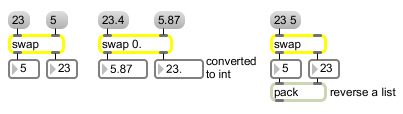# swap

Reverse the sequential order of two floating-point numbers

## Description

swap swaps the values of its inlets, preserving right-to-left ordering. The first outlets (and second inlets) type follow the argument (or is an int if you give no argument). The second outlet's (and first inlets) type is int or float depending on whether you use the name "swap" or "fswap".

## Arguments

Name Type Opt Description
left-initial-value int or float opt Sets an initial value for the number which is to be sent out the left outlet. If there is no argument, the initial value is 0. If there is an int argument or no argument, an int is sent out the left outlet. (The number sent out the right outlet is always a float.)

## Messages

 bang In left inlet: Swaps and sends out the numbers currently stored in fswap. int input [int] If there is a float argument, the numbers are converted to float. If there is an int argument or no argument, the number received in the right inlet is stored as an int. (inlet1) input [int] In right inlet: The number is stored to be sent out the left outlet when a number is received in the left inlet. float input [float] In left inlet: The number is sent out the right outlet, then the number in the right inlet is sent out the left outlet. In right inlet: The number is stored to be sent out the left outlet when a number is received in the left inlet. (inlet1) input [float] In right inlet: The number is stored to be sent out the left outlet when a number is received in the left inlet.

## Output

float: The number sent out the right outlet is always a float. The number sent out the left outlet is a float only if there is a float argument.
int: When a number is received in the left inlet, the number in each inlet is sent out the opposite outlet. If there is an int argument or no argument, an int is sent out the left outlet.

## ExamplesNumbers are sent out in reverse order from the order in which they were received